Average sample number

ASN

A term occurring in the area of statistics called sequential analysis. In sequential procedures for statistical estimation, hypothesis testing or decision theory, the number of observations taken (or sample size) is not pre-determined, but depends on the observations themselves. The expected value of the sample size of such a procedure is called the average sample number. This depends on the underlying distribution of the observations, which is often unknown. If the distribution is determined by the value of some parameter, then the average sample number becomes a function of that parameter.

Ifdenotes the sample size andis the unknown parameter, then the average sample number is given by(a1)

Some aspects of the average sample number in the case where the observations are independent Bernoulli random variableswith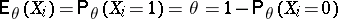are given below.

Consider the case of the curtailed version of a test of the hypotheses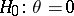versusthat takesobservations and decidesif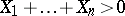(cf. also Statistical hypotheses, verification of). The curtailed version of this test is sequential and stops the first time thator at time, whichever comes first. Then the average sample number is given by(a2)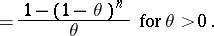This formula is a special case of Wald's lemma (see [a3] and Wald identity), which is very useful in finding or approximating average sample numbers. Wald's lemma states that ifare independent random variables with common expected value (cf. also Random variable) and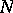is a stopping time (i.e.,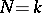is determined by the observations) with finite expected value, then letting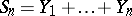,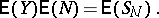(a3)

Thus, for, one has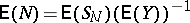.

In this example,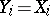,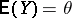, and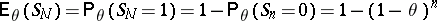. The average sample number then follows from (a3) and agrees with (a2). See [a1] for asymptotic properties of the average sample number for curtailed tests in general.

In testingversus, the logarithm of the likelihood ratio (cf. also Likelihood-ratio test) afterobservations is easily seen to be of the form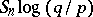, where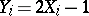. Thus, if, the sequential probability ratio test stops the first time that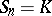and decidesor the first time that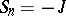and decides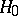for positive integers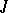and. In this case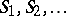is a random walk taking steps to the right with probabilityand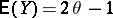in formula (a3). Thus, if, the average sample number is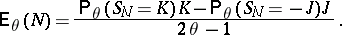(a4)

Well-known formulas from the theory of random walks show that, where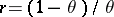.

If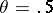, this method fails. One must then use another result of A. Wald, stating that if, but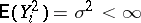, then(a5)

In this example, for, one has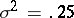and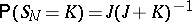. Then (a5) yields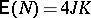.

In order to decide which of two sequential tests is better, it is important to be able to express the average sample number in terms of the error probabilities of the test. Then the average sample numbers of different tests with the same error probabilities can be compared. In this example, the probability of a type-I error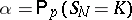and the probability of a type-II error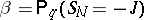(cf. also Error). From this one sees thatand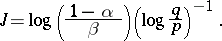In particular, it follows that if, then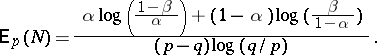(a6)

This formula and the analogous one with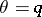are often used to measure the efficiency of the sequential probability ratio test against other sequential tests with the same error probabilities

Similar formulas can be found for sequential probability ratio tests for sequences of independent random variables with other distributions, but in general the formulas are approximations since the tests will stop when the logarithm of the likelihood ratio first crosses the boundary rather than when it first hits the boundary. Many studies (see [a2]) consider the adequacy of approximations for the average sample number of sequential probability ratio tests and some other sequential tests whose stopping times are more complicated. In areas such as signal detection, average sample numbers are also studied for sequential tests where the observations are not independent, identically distributed random variables.